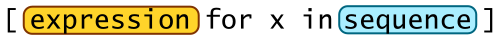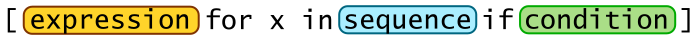# List comprehensions

By Martin McBride, 2018-08-10
Tags: comprehension list comprehension
Categories: python language intermediate pythonDeclaring lists in Python is quite simple:

k = [0, 1, 2, 3]


But what if you needed a much longer list, where the elements followed some pattern, for example a list of all the numbers from 0 to 999?

You would probably use a list similar to this

k = []
for x in range(1000):
k.append(x)


This code starts with an empty list, and the loop appends values 0, 1, 2 ... 999, one by one.

List comprehensions offer a shorthand way of doing this. Here is the same code written as a list comprehension:

k = [x for x in range(1000)]


As you can see, all the elements of the loop are there, just shortened an re-arranged a bit. The easiest way to understand it is to read it as an English sentence:

The elements of k are the values of x for every x in the range 0 to 999


You can also create a list based on some calculation using the value x, for example:

k = [x*x for x in range(1000)]


Fills the list with successive squared values (the final value is 999*999):

[0, 1, 4, 9, 16 ... 998001]


You don't have to use a range function, you can use any sequence, including another list:

a = [2, 3, 1, 5]
b = [x + 2 for x in a]


This adds 2 to each value in a, to create the corresponding value in b.

[4, 5, 3, 7]


Here is the general form for a simple list comprehension:Where expression might be something like x + 2, and sequence might be a range, list, or in general any iterable. For each value x in the sequence, the expression is evaluated and the result added to the list.

A list comprehension is obviously a bit shorter than the equivalent loop, but it has other advantages:

• It is more readable (when you get used to it).
• The intent is clear - to initialise a list. A loop could be doing anything, you have to read it to check that it doesn't have any other side effects. A list comprehension just sets up the list, you know it isn't up to anything else.
• For that reason, it is less error prone.
• It is a Python expression, so you can incorporate it into other statements to keep you code short and readable.

## Using conditions

Suppose we have an existing list of numbers, and we want to create a second list which excludes all the negative numbers. We might use this loop:

x = [1, -2, 4, 5, -3, -9, 0]
y = []
for n in x:
if n >= 0:
y.append(n)


Here, we check the value of n, and only append it to list y if it is not negative. But here is an alternative - we can use a list comprehension:

x = [1, -2, 4, 5, -3, -9, 0]
y = [n for n in x if n >= 0]


If you are starting to get used to list comprehensions, you might see that not only is this code shorter, it is also more readable.

As another example, let's find a list of square numbers which end in 6. Remember you can find the last digit of a number using modulo (%) 10. Here is the loop:

k = [x*x for x in range(1000) if x*x % 10 == 6]


Which gives

[16, 36, 196, 256, 576 ...


Here is the general form for a conditional list comprehension:Where expression might be something like x*x, condition sequence is an iterable, and condition could be something like x >= 0. For each value x in the sequence, the condition is checked, and if it is true the expression is evaluated and the result added to the list.

Finally, just to show that it doesn't always have to be about numbers, let's extract a list of colour names which start with vowels (and, yes, I have picked some of the sillier ones from the CSS colour names):

u = ['aliceblue',
'bisque',
'dodgerblue',
'oldlace',
'papayawhip',
'olivedrab']
v = [s for s in u if s in 'aeiou']


In this case, the condition is :

s in 'aeiou'


giving the result:

['aliceblue', 'oldlace', 'olivedrab']


## Creating 2D lists

What if the expression part of the comprehension returns a list itself? Such as this:

k = [*4 for x in range(3)]


Well, this creates a list with 3 elements, where each element is a list [0, 0, 0, 0].

Remember, *4 gives [0, 0, 0, 0]

This creates a 2D list:

[[0, 0, 0, 0],
[0, 0, 0, 0],
[0, 0, 0, 0],
[0, 0, 0, 0]]


This is actually quite a useful recipe for creating 2D lists. The following doesn't work:

k = [*4]*3   #Doesn't work!
k = 3
print(k)        #[[0, 0, 3, 0], [0, 0, 3, 0], [0, 0, 3, 0]] Ooops!


This code doesn't work because it only creates the inner list once. The outer list contains 3 copies of the same inner list.

Now we can go a stage further, and use a second list comprehension as our expression:

k = [[x + y for y in range(3)] for x in range(3)]


Here is what it does:

• The x loop runs 3 times, and on each pass through, the inner list comprehension executes.
• The inner list comprehension creates a list of 3 elements with a value equal to y plus the current value of x.
• The result is a 3 by 3 list where each element is equal to the sum of its column and row numbers.

[[0, 1, 2], [1, 2, 3], [2, 3, 4]]

Try these next two, and check what happens:

u = [[1 if x == y else 0 for y in range(3)] for x in range(3)]
v = [[0 for y in range(x + 1)] for x in range(3)]


Here are a few variants you can look up if you are interested, which aren't cover here because they aren't as common:

• Set comprehensions - like list comprehensions but they create a set (just use {} instead of []).
• Dictionary comprehensions
• Using nested for loops (two for loops to create a 1 dimensional list). Some people feel this is a step too far, and isn't really as readable as just using explicit loop code.

In addition, the built-in functions map and filter can do a similar job. They are less concise, but more flexible. It is often a matter of personal preference.